# 雾天中车牌识别系统的设计与实现Design and Implementation of License Plate Recognition System in Fog

• 全文下载: PDF(972KB)    PP.985-992   DOI: 10.12677/CSA.2019.95111
• 下载量: 278  浏览量: 3,751

1. 引言

2. 图像去雾技术

1) 灰暗的图像的直方图中主要成分集中在像素值较小的一侧；

2) 明亮的图像的直方图中主要成分倾向于像素值较大的一侧；

3) 对比度低的图像的直方图所占灰度级较少且主要成分集中于中部的灰度级；

4) 对比度高的图像的直方图所占灰度级较多且主要成分分布均匀。

$s=T\left(r\right)$ (1)

1) 在 $0\le r\le 1$ 区间内， $s=T\left(r\right)$ 值为单调增加。

2) 对于 $0\le r\le 1$ ，有 $0\le s=T\left(r\right)\le 1$

$r={T}^{-1}\left(s\right),0\le s\le 1$ (2)

1) 根据分布函数的定义可以求得随机变量s的分布函数 ${F}_{s}\left(s\right)$ ，即

$F\left(s\right)={\int }_{-\infty }^{r}{P}_{r}\left(x\right)\text{d}x$ (3)

2) 根据分布函数与概率密度函数之导数关系，可得出 ${P}_{s}\left(s\right)$ ，即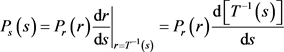(4)

3. 车牌识别技术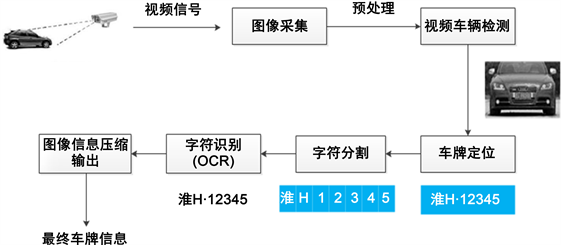Figure 1. Basic steps for license plate recognition

3.1. 图像预处理

1) 图像的预处理是整个图像识别的前提，因为计算机不能像人眼一样可以很直观的观测到整体的图像，而是通过输入图像的每个像素点的具体值，以及这些像素点之间的排列关系来识别图像。所以在做计算机视觉的过程中，我们需要先对图片进行一系列的预处理过程，好让计算机或者是程序算法更好的理解图片内容的含义  ，依据不同方法实现图像视感品质的提高，尽可能还原较高真实度的信息，并且提高相关信息的可检测性，提高提取及识别的可靠程度，以方便下一步的处理。具体的处理步骤主要有图像灰度图的转化、边缘检测、腐蚀、平滑处理以及移除最小对象等  。

① 图像灰度图的转化

$H=0.299R+0.587G+0.114B$ (1)

$H=\frac{B+G+R}{3}$ (2)

② 图像的边缘检测

③ 灰度图的腐蚀

④ 平滑处理

⑤ 移除小对象

3.2. 车牌定位

3.3. 字符分割

3.4. 字符识别

4. 实验结果及分析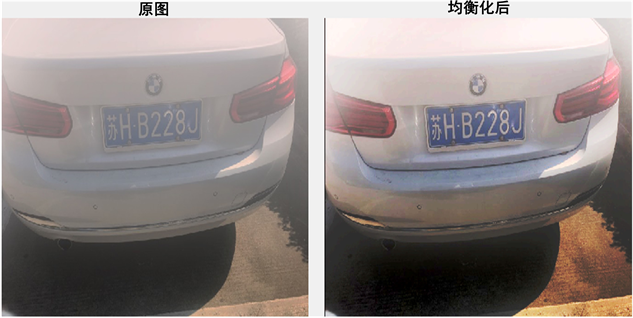Figure 2. Image before and after defogging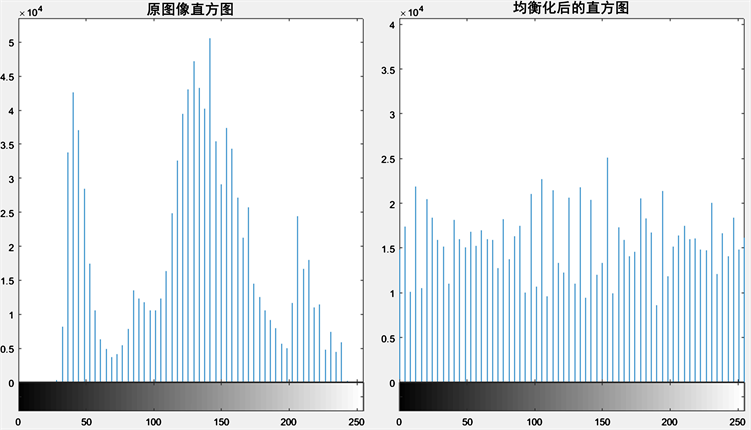Figure 3. Histogram comparison after equalization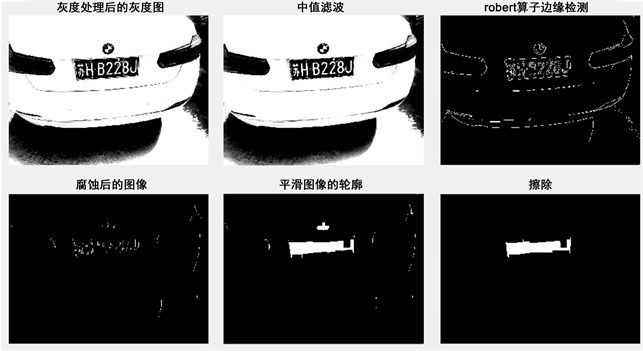Figure 4. Image preprocessing process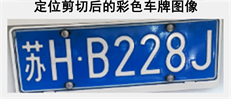Figure 5. Image of the license plate accurately positioned after pre-processing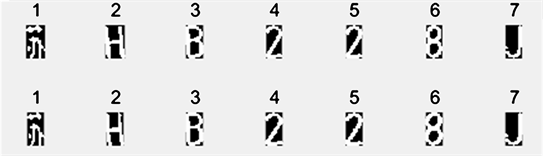Figure 6. Accurately segmenting license plate characters

5. 结语

NOTES

*通讯作者。

  莫玲. 图像处理技术在车牌识别中的研究[J]. 轻工科技, 2018, 34(8): 88-90.  黄辰阳. 基于图像处理的车牌识别方法研究[D]: [硕士学位论文]. 广州: 广东工业大学, 2018.  张莉莉, 武艳. 基于MATLAB的车牌识别系统的设计仿真[J]. 数字技术与应用, 2017(10): 127-128.  Zhuang, J.F., Hou, S.H., Wang, Z.L. and Zha, Z.J. (2018) Towards Human-Level License Plate Recognition. Springer, 314-329. https://doi.org/10.1007/978-3-030-01219-9_19  钟彩, 杨兴耀. 车牌定位与车牌分割技术研究[J]. 电脑知识与技术, 2018, 14(2): 172-173.  陈天华. 数字图像处理及应用[M]. 北京: 清华大学出版社, 2019.  苏祺航. 车牌识别系统的研究[J]. 电子制作, 2018(21): 55-57.  董恒祎. 基于图像处理的车牌识别检测系统[J]. 通讯世界, 2018(8): 269-271.